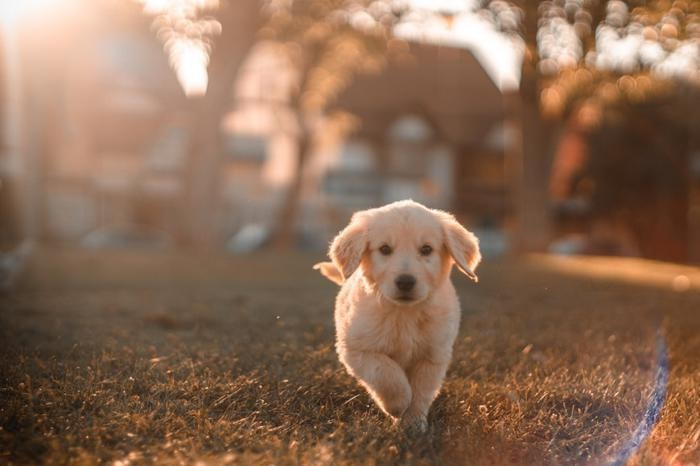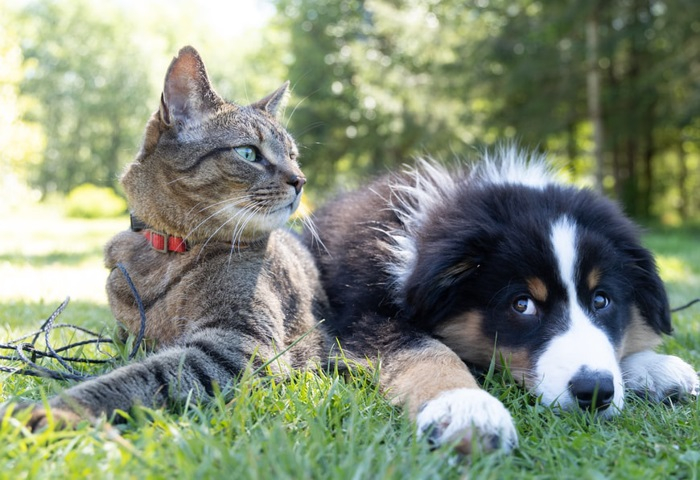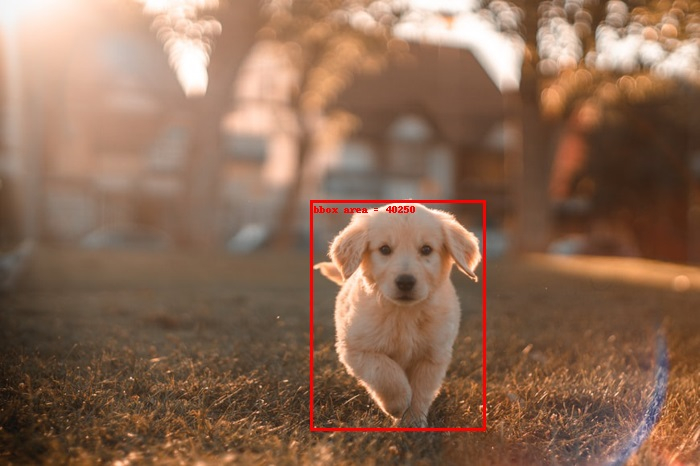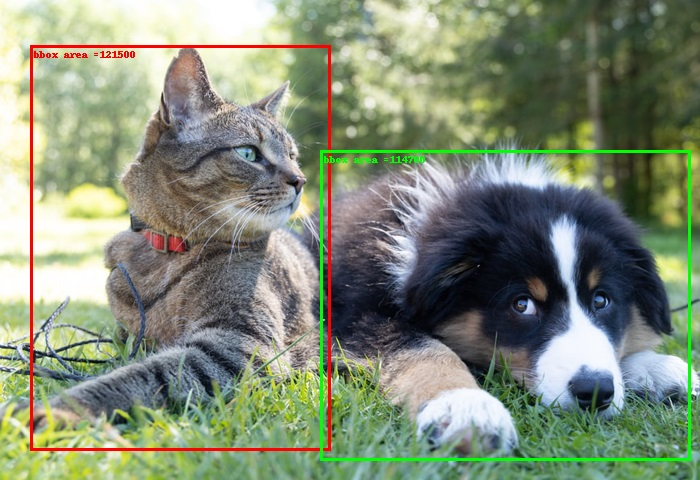# How to compute the area of a set of bounding boxes in PyTorch?

The torchvision.io package provides functions to perform different IO operations. To compute the area of a bounding box or a set of bounding boxes, torchvision.io package provides the box_area() function. This function takes the bounding boxes as an input parameter and returns the area of each box.

The bounding boxes should be torch Tensors of size [N,4] where N is the number of bounding boxes for which the area to be calculated. Each bounding box is specified by the coordinate (xmin, ymin, xmax, ymax). In other words − 0 ≤ xmin < xmax, and 0 ≤ ymin < ymax. The computed area is a torch Tensor of size [N].

To compute the area of a single bounding box, we unsqueeze the bounding box tensor to make it a two-dimension tensor.

## Syntax

torchvision.ops.box_area(boxes)

## Parameters

• boxes - A [N,4] torch tensor containing the bounding boxes. Each bounding box is expected in (xmin, ymin, xmax, ymax) format where 0 ≤ xmin < xmax, and 0 ≤ ymin < ymax.

## Output

It returns a torch Tensor of size [N] with the areas of bounding boxes.

## Steps

• Import the required libraries. In all the following examples, the required Python libraries are torch and torchvision. Make sure you have already installed them.

import torch
import torchvision
from torchvision.utils import draw_bounding_boxes
from torchvision.ops import box_area
• Read a JPEG or PNG image using image_read() function. Specify the full image path with image types (.jpg or .png). The output of this function is a torch tensor of size [image_channels, image_height, image_width].

img = read_image('dog.png')
• Define the bounding box as a torch tensor. The bounding box tensor should be of dtype torch.int. Unsqueeze the tensor if the area of only one bounding box is to be calculated.

bbox = (310, 200, 485, 430)
# convert the bbox to torch tensor
bbox = torch.tensor(bbox, dtype=torch.int)
• Compute the area bounding box using box_area(). Optionally, assign the image with the bounding box drawn to a new variable.

area = box_area(bbox)
• Draw a bounding box on the image using the draw_bounding_boxes() function. We put the computed area inside the bounding box as a label.

labels= [f"bbox area = {area.item()}"]
img=draw_bounding_boxes(img, bbox, labels= labels, width=3,colors=(255,255,0))
• Convert the image tensor to a PIL image and display it.

img = torchvision.transforms.ToPILImage()(img)
img.show()

## Input Images

We will use these images as the input files in the following examples.## Example 1

In the following Python program, we compute the area of a single bounding box and put this area as a label on the image and display the image.

# Import the required libraries
import torch
import torchvision
from torchvision.utils import draw_bounding_boxes
from torchvision.ops import box_area

# bounding box in (xmin, ymin, xmax, ymax) format
# top-left point=(xmin, ymin), bottom-right point = (xmax, ymax)bbox = (310, 200, 485, 430)

# convert the bbox to torch tensor
bbox = torch.tensor(bbox, dtype=torch.int)
print(bbox)
print(bbox.size())

# unsqueeze the bbox to make it 2D
bbox = bbox.unsqueeze(0)
print(bbox.size())

# Compute the bounding box area
area = box_area(bbox)
print("BBOX area:", area)
labels= [f"bbox area = {area.item()}"]
img=draw_bounding_boxes(img, bbox, labels= labels, width=3,colors=(255,255,0))

# b=a.permute(1,2,0)
# plt.imshow(b)
# plt.show()
img = torchvision.transforms.ToPILImage()(img)
img.show()

## Output

tensor([310, 200, 485, 430], dtype=torch.int32)
torch.Size()
torch.Size([1, 4])
BBOX area: tensor(, dtype=torch.int32)## Example 2

In the following Python program, we compute the area of a set of two bounding boxes and put the areas as labels on the image and display the image.

import torch
from PIL import Image
import torchvision
from torchvision.utils import draw_bounding_boxes
from torchvision.ops import box_area

# bounding box in (xmin, ymin, xmax, ymax) format
bbox1 = [30, 45, 330, 450]
bbox2 = [320, 150, 690, 460]
bbox = [bbox1, bbox2]
bbox = torch.tensor(bbox, dtype=torch.int)
print(bbox)
print(bbox.size())

area = box_area(bbox)
labels = [f"bbox area ={a}" for a in area]
print(labels)
img=draw_bounding_boxes(img, bbox, labels = labels, width=3,colors=[(255,0,0),(0,255,0)])
img = torchvision.transforms.ToPILImage()(img)
img.show()

## Output

tensor([[ 30, 45, 330, 450],
[320, 150, 690, 460]], dtype=torch.int32)
torch.Size([2, 4])
['bbox area =121500', 'bbox area =114700']